# How to use pandas cut() and qcut()?

• Difficulty Level : Hard
• Last Updated : 30 Jun, 2022

Pandas is an open-source library that is made mainly for working with relational or labeled data both easily and intuitively. It provides various data structures and operations for manipulating numerical data and time series.

In this tutorial, we’ll look at pandas’ intelligent cut and qcut functions. Basically, we use cut and qcut to convert a numerical column into a categorical one, perhaps to make it better suited for a machine learning model (in case of a fairly skewed numerical column), or just for better analyzing the data at hand. Now, rather than blurting out technical definitions of cut and qcut, we’d be better off seeing what both these functions are good at and how to use them.

We’ll first import the necessary data manipulating libraries.

## Python3

 `import` `pandas as pd``import` `numpy as np``import` `matplotlib.pyplot as plt`

We’ll be using the CarDekho dataset, containing data about used cars listed on the platform. You can find the dataset here

• ‘Year’ is the year in which the car was purchased.
• ‘Selling_Price’ is the price the owner wants to sell the car at.
• ‘Present_Price’ is the current ex-showroom price of the car.
• ‘Owner’ defines the number of owners the car has previously had, before this car was put up on the platform.

Rest of the columns are pretty self explanatory.

## Python3

 `df ``=` `pd.read_csv(``'cardekho.csv'``)``df.head()`

Output: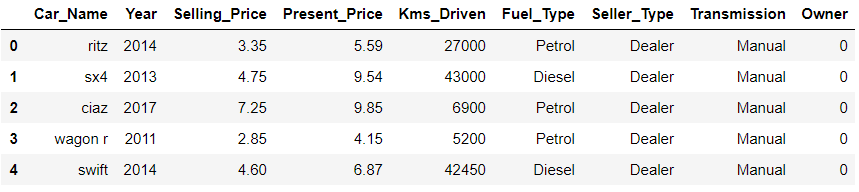## Python3

 `# no missing values in the dataset``df.info()`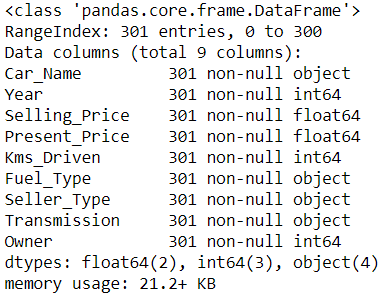We’ll infuse a missing value to better demonstrate how cut and qcut would handle an ‘imperfect’ dataset.

## Python3

 `df.loc[``0``, ``'Year'``] ``=` `np.nan` `# these are the 'unique' years in``# the data``np.array(``sorted``(df.Year.unique()))`

Output: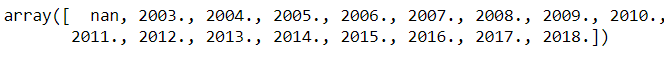## pd.cut()

We can use the ‘cut’ function in broadly 2 ways: by specifying the number of bins directly and let pandas do the work of calculating equal-sized bins for us, or we can manually specify the bin edges as we desire.

## Python3

 `# right = True, by default``pd.cut(df.Year, bins``=``3``, right``=``True``).head()`

Output: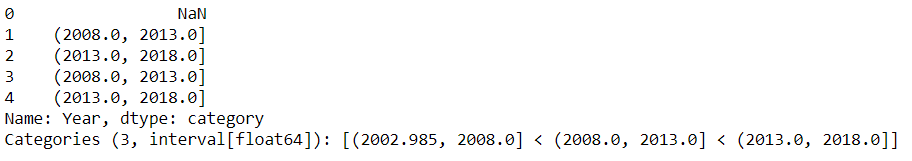When we specified bins=3, pandas saw that the year range in the data is 2003 to 2018, hence appropriately cut it into 3 equal-width bins of 5 years each: [(2002.985, 2008.0] < (2008.0, 2013.0] < (2013.0, 2018.0]. Note that pandas automatically took the lower bound value of  the first category (2002.985) to be a fraction less that the least value in the ‘Year’ column (2003), to include the year 2003 in the results as well, because you see, the lower bounds of the bins are open ended, while the upper bounds are closed ended (as right=True)

Note: Did you notice that the NaN values are kept as NaNs in the output result as well? When we specify right=False, the left bounds are now closed ended, while right bounds get open ended.

## Python3

 `pd.cut(df.Year, bins``=``3``, right``=``False``).head()`

Output: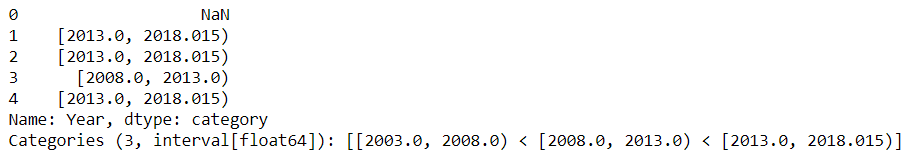Instead of getting the intervals back, we can specify the ‘labels’ parameter as a list for better analysis.

## Python3

 `pd.cut(df.Year, bins``=``3``,``       ``labels``=``[``'old'``, ``'medium'``, ``'new'``]).head()`

Output: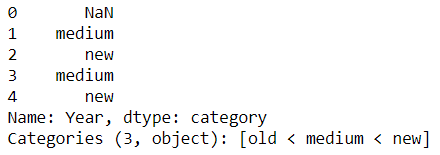We will assign this series back to the original dataframe:

## Python3

 `df[``'Yr_cut'``] ``=` `pd.cut(df.Year, bins``=``3``,``                      ``labels``=``[``'old'``, ``'medium'``, ``'new'``])``df.head()`

Output: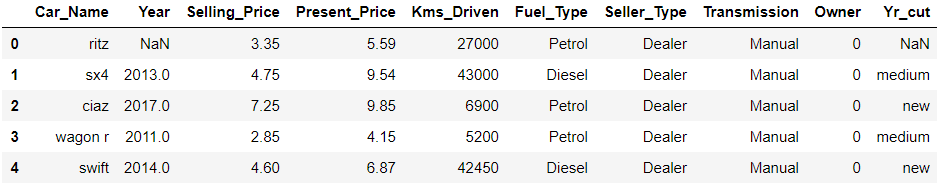If we specify labels=False, instead of bin labels, we will get numeric representation of the bins: Here, 0 represents old, 1 is medium and 2 is new.

## Python3

 `pd.cut(df.Year, bins``=``3``, labels``=``False``).head()`

Output: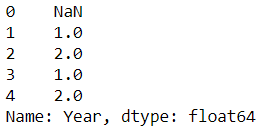Just to see how many values fall in each bin:

## Python3

 `df[``'Yr_cut'``].value_counts()`

Output: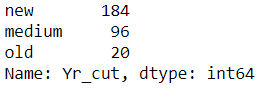And just because drawing a graph pleases more people than offends..

## Python3

 `df[``'Yr_cut'``].value_counts().plot(kind``=``'barh'``)``plt.show()`

Output: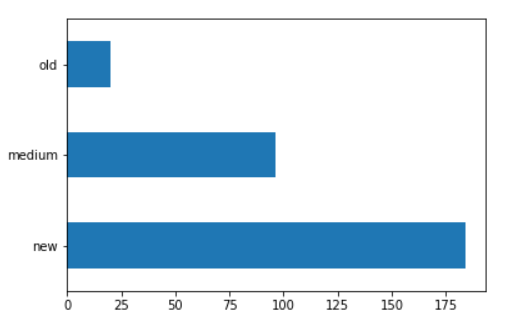Now, if we need the bin intervals along with the discretized series at one go, we specify retbins=True. Pandas will give us back a tuple containing 2 elements: the series, and the bin intervals. We will use tuple unpacking to grab both outputs.

## Python3

 `cut_series, cut_intervals ``=` `pd.cut(df.Year,``                                   ``bins``=``3``,``                                   ``retbins``=``True``)` `print``(``"Cut series:"``)``print``(cut_series.head())``print``()``print``(``"Cut intervals: "``, cut_intervals)`

Output: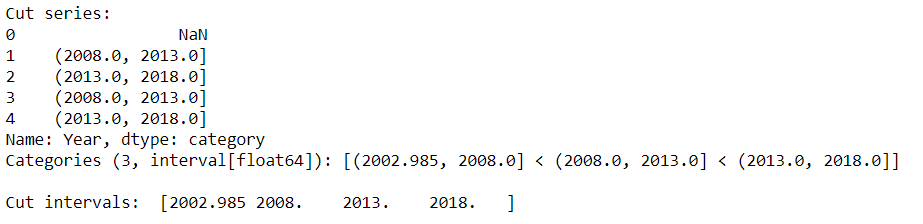As mentioned earlier, we can also specify bin edges manually by passing in a list:

## Python3

 `pd.cut(df.Year, bins``=``[``2003``,``                      ``2007``,``                      ``2010``,``                      ``2015``,``                      ``2018``],``       ``include_lowest``=``True``).head()`

Output: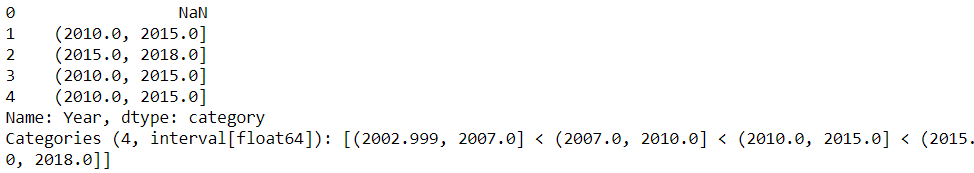Here, we had to mention include_lowest=True. Can you guess why? Because by default ‘include_lowest’ parameter is set to False, and hence when pandas sees the list that we passed, it will exclude 2003 from calculations. For the eagle-eyed, we could have used any value less than 2003 as well, like 1999 or 2002 or 2002.255 etc and gone ahead with the default setting of include_lowest=False.

## pd.qcut()

Qcut (quantile-cut) differs from cut in the sense that, in qcut, the number of elements in each bin will be roughly the same, but this will come at the cost of differently sized interval widths. On the other hand, in cut, the bin edges were equal sized (when we specified bins=3) with uneven number of elements in each bin or group. Also, cut is useful when you know for sure the interval ranges and the bins,

For example, if binning an ‘age’ column, we know infants are between 0 and 1 years old, 1-12 years are kids, 13-19 are teenagers, 20-60 are working class grownups, and 60+ senior citizens. So we can appropriately set bins=[0, 1, 12, 19, 60, 140] and labels=[‘infant’, ‘kid’, ‘teenager’, ‘grownup’, ‘senior citizen’]. In qcut, when we specify q=5, we are telling pandas to cut the Year column into 5 equal quantiles, i.e. 0-20%, 20-40%, 40-60%, 60-80% and 80-100% buckets/bins.

## Python3

 `pd.qcut(df.Year, q``=``5``).head(``7``)`

Output: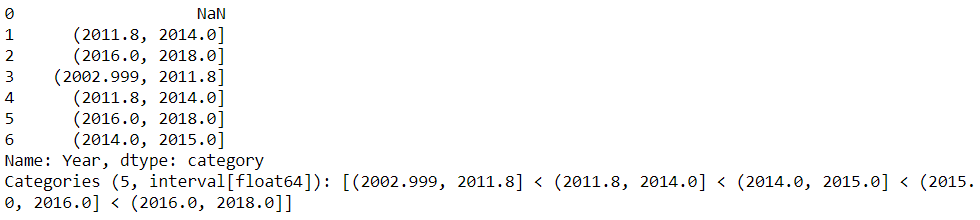We’ll assign this series to the dataframe.

## Python3

 `df[``'Yr_qcut'``] ``=` `pd.qcut(df.Year, q``=``5``,``                        ``labels``=``[``'oldest'``,``                                ``'not so old'``,``                                ``'medium'``,``                                ``'newer'``,``                                ``'latest'``])``df.head()` `df[``'Yr_qcut'``].value_counts().plot(kind``=``'barh'``)``plt.show()` `qcut_series, qcut_intervals ``=` `pd.qcut(df.Year, q``=``5``,``                                      ``labels``=``[``'oldest'``,``                                              ``'not so old'``,``                                              ``'medium'``,``                                              ``'newer'``,``                                              ``'latest'``],``                                      ``retbins``=``True``)` `qcut_series.value_counts()`

Output: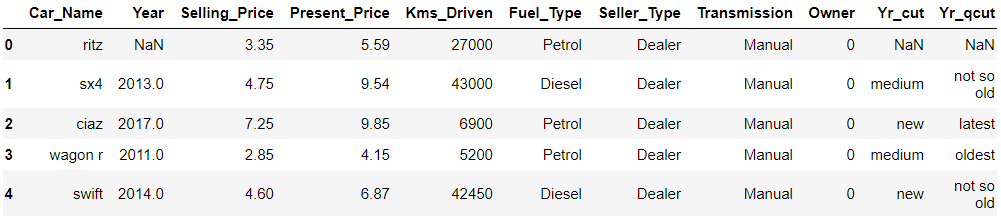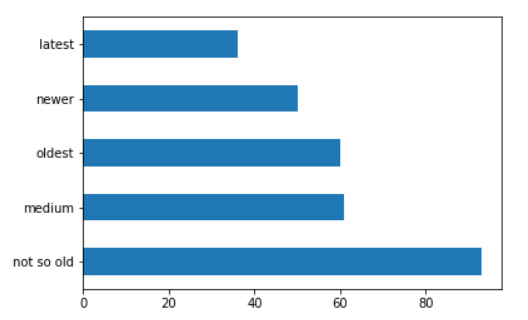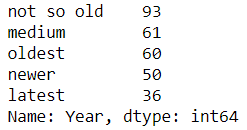Now just to highlight the fact that q=5 indeed implies splitting values into 5 equal quantiles of 20% each, we’ll manually specify the quantiles, and get the same bin distributions as above.

## Python3

 `pd.qcut(df.Year,``        ``q``=``[``0``, ``0.2``, ``0.4``,``           ``0.6``, ``0.8``, ``1.0``],``        ``labels``=``[``'oldest'``,``                ``'not so old'``,``                ``'medium'``,``                ``'newer'``,``                ``'latest'``]).value_counts()`

Output: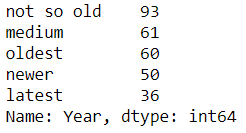We’ll now see the qcut intervals array we got using tuple unpacking:

## Python3

 `qcut_intervals`

Output: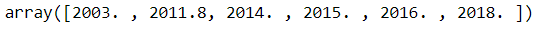You see? Here in qcut, the bin edges are of unequal widths, because it is accommodating 20% of the values in each bucket, and hence it is calculating the bin widths on its own to achieve that objective.

## Python3

 `# demonstrating with some random quantiles``pd.qcut(df.Year, q``=``[``0``,``0.15``,``0.35``,``                    ``0.51``,``0.78``,``1``]).head()`

Output: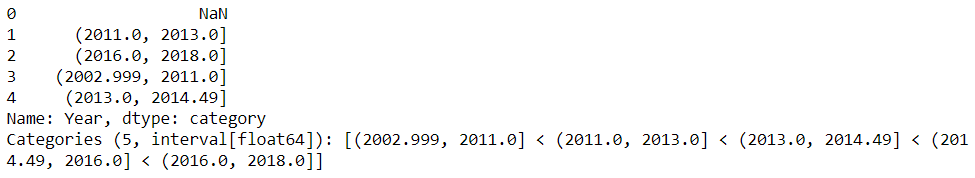Now it is binning the data into our custom made list of quantiles of 0-15%, 15-35%, 35-51%, 51-78% and 78-100%.
With qcut, we’re answering the question of “which data points lie in the first 15% of the data, or in the 51-78 percentile range etc. Additionally, we can also use pandas’ interval_range, or numpy’s linspace and arange to generate a list of interval ranges and feed it to cut and qcut as the bins and q parameter respectively.

## Python3

 `pd.cut(df.Year,``       ``bins``=``pd.interval_range(start``=``2002.99``,``                              ``end``=``2018``,``                              ``periods``=``3``)).head()`

Output: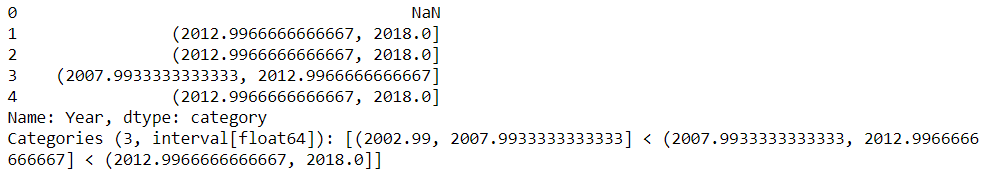Using linspace:

## Python3

 `# basically dividing data into 3``# quantiles``pd.qcut(df.Kms_Driven,``        ``q``=``np.linspace(``0``,``1``,``4``)).head()`

Output: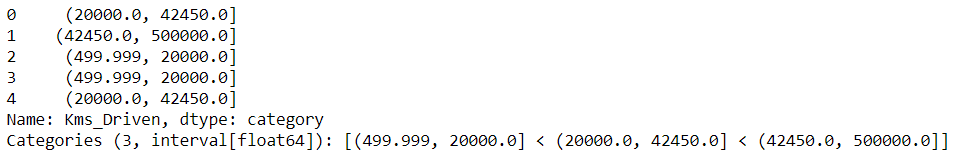Using arange:

## Python3

 `pd.qcut(df.Selling_Price,``        ``q``=``np.arange(``0``, ``1.01``, ``0.25``),``        ``labels``=``[``'inexpensive'``,``                ``'average'``,``                ``'high cost'``,``                ``'over budget'``]).head()`

Output: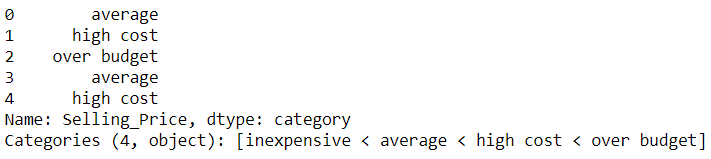Sometimes when we ask pandas to calculate the bin edges for us, you may run into an error which looks like:
ValueError: Bin edges must be unique error. This implies that while calculating the bin intervals, pandas found that some bin edges were the same on both ends, like an interval of (2014, 2014] and hence it raised that error. This usually happens when the number of bins is large and the value range of the particular column is small. You can silence this error by passing the argument of duplicates=’drop’.

My Personal Notes arrow_drop_up